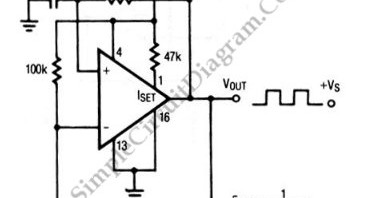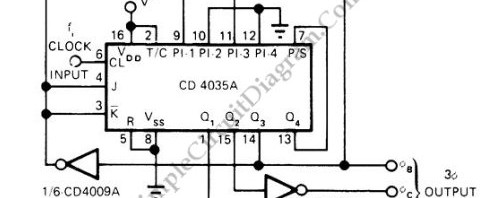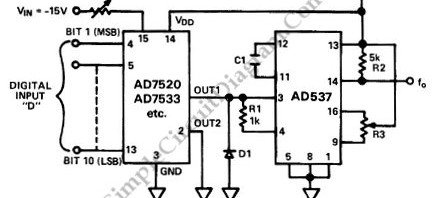## Square Wave Oscillator using Comparator/Op-AmpThe circuit shown in this schematic diagram is a square-wave oscillator circuit.  The main component of this oscillator circuit is LP165/365 comparator. As timing component, it uses capacitor and resistor to determine the frequency operation, generating square waves signal. Here is the schematic diagram of the circuit: We can change the values of R and C to produce different frequencies. […]

## Pulse Generator Produces 3-Phase OutputThis is a schematic diagram of a three-phase pulse generator circuit. This circuit produce 3 phase overlapped output similar with 3 phase  AC powerline, you can say this circuit produce the DC pulse version. This schematic diagram shows two CMOS inverters and CMOS 4-bit shift register as the circuit’s  main components. The shift register is configured as divide-by-6 Johnson counter, […]

## Starting Network for Astable MultivibratorA flip-flop that generates two unstable states (high-low) is called astable multivibrator, a fancy name for you to say it’s an oscillator. The very basic of such circuit consist of two transistor where the collector of each transistor is wired in such way they try to short the base of the other transistor through a capacitor. At the beginning of […]